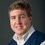# My answer to thinking like a theorist (F=ma version)Note by David Mattingly
6 years, 1 month ago

MarkdownAppears as
*italics* or _italics_ italics
**bold** or __bold__ bold
- bulleted- list
• bulleted
• list
1. numbered2. list
1. numbered
2. list
Note: you must add a full line of space before and after lists for them to show up correctly
paragraph 1paragraph 2

paragraph 1

paragraph 2

[example link](https://brilliant.org)example link
> This is a quote
This is a quote
    # I indented these lines
# 4 spaces, and now they show
# up as a code block.

print "hello world"
# I indented these lines
# 4 spaces, and now they show
# up as a code block.

print "hello world"
MathAppears as
Remember to wrap math in $ ... $ or $ ... $ to ensure proper formatting.
2 \times 3 $2 \times 3$
2^{34} $2^{34}$
a_{i-1} $a_{i-1}$
\frac{2}{3} $\frac{2}{3}$
\sqrt{2} $\sqrt{2}$
\sum_{i=1}^3 $\sum_{i=1}^3$
\sin \theta $\sin \theta$
\boxed{123} $\boxed{123}$

## Comments

Sort by:

Top Newest

excellent solution. Hats off!

- 6 years, 1 month ago

Log in to reply

i can't talk to much, but you are give us a great problem every weeks ,i never got this lesson on my school, thank you sir, im agree with Siddharth , that amazing solution :)

- 6 years, 1 month ago

Log in to reply

we can have F=ma only when we azzume that mass is constant but for every partical mass can't be constant as newtons first law say that F = d/dt(mv) the on differnsiate it we get F = (m)(d/dt(v))+(v)(d/dt(m)) so it says that if your mass is constant than d/dt(m)=0 and you will get F=ma but if it is not constant than you will get the above equation which is the foundation of einstian most famous equation E = m(c^2)

- 6 years, 1 month ago

Log in to reply

can we move the n relative to an infinite number????

- 6 years, 1 month ago

Log in to reply

About your F=mv case, can you elaborate on how it doesnt hold up to relativity? If F=mv and we at the same time insist that there is a universal "at rest", that means that we can introduce fictive forces to moving reference frames, much the same way we do in the current Newtonian model for accelerating reference frames. I am not physicist enough to be able to find problematic conclusions with this approach. Conclusions that do not match well with experiments and empirical evidence, yes, but not intrinsically problematic conclusions.

- 6 years, 1 month ago

Log in to reply

But you can't specify a universal at rest and maintain relativity.

Staff - 6 years, 1 month ago

Log in to reply

Why move to relativity for n=1? Let us take F=mv, v is velocity. Let us also take F as the external force on the particle. Now, if F=0, it must mean that v=0. However, if only this were true, we would have to modify Newton's first law to only "An object at rest continues to remain at rest". But then what about the case of uniform velocity? Our new law will imply that in case an object travels with uniform velocity, it will have some constant force acting on it, which I think is wrong, since it is experimentally verified that objects travelling with uniform velocity must have zero external force acting on them (Take as an approximate example, a linear air track).

- 6 years, 1 month ago

Log in to reply

F=0 it isn't have to be that v=0. And, m times v is a momentum not a force.

- 6 years, 1 month ago

Log in to reply

1 time derivation of position is velocity.it tells you with respect to time how rapidly are u scanging the dispalcement. the derivative of velocity with respect to time is called as acceleration.it tells me how rapidly i am changing my velocity. so first derivative will give you velocity and then derivative again of velocity is acceleration.thus there are two time derivatives involved.first will make position as velocity and second will make it as acceleration. infact even third derivative also exists,its called as jerk.......

- 6 years, 1 month ago

Log in to reply

×

Problem Loading...

Note Loading...

Set Loading...